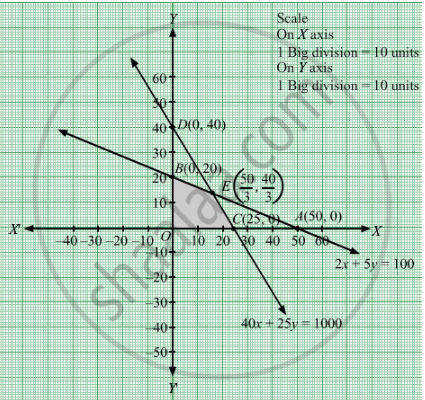# If a Young Man Drives His Vehicle at 25 Km/Hr, He Has to Spend Rs 2 per Km on Petrol. If He Drives It at a Faster Speed of 40 Km/Hr, the Petrol Cost Increases to Rs 5/Per Km. - Mathematics

Sum

If a young man drives his vehicle at 25 km/hr, he has to spend Rs 2 per km on petrol. If he drives it at a faster speed of 40 km/hr, the petrol cost increases to Rs 5/per km. He has Rs 100 to spend on petrol and travel within one hour. Express this as an LPP and solve the same.

#### Solution

Let young man drives km at a speed of  25 km/hr  and y km at a speed of  $40 km/hr$  Clearly, $x, y \geq 0$
It is given that, he spends Rs 2 per km if he drives at a speed of $25 km/hr$  and Rs 5 per km if he drives at a speed of $40 km/hr$  . Therefore, money spent by him when he travelled x km and y km is Rs 2x and Rs 5respectively.
It is given that he has a maximum of Rs 100 to spend.
Thus, $2x + 5y \leq 100$

$\text{ Time spent by him when travelling with a speed of 25 km/hr } = \frac{x}{25}hr$
$\text{ Time spent by him when travelling with a speed of 40 km/hr} = \frac{x}{40}hr$

Also, the available time is of 1 hour.

$\frac{x}{25} + \frac{y}{40} \leq 1$
$\Rightarrow 40x + 25y \leq 1000$

The distance covered is Z = $x + y$ which is to be maximised.
Thus, the mathematical formulat​ion of the given linear programmimg problem is
Max Z = $x + y$
subject to

$2x + 5y \leq 100$

$40x + 25y \leq 1000$

$x, y \geq 0$

First we will convert inequations into equations as follows:
2x + 5y = 100, 40x + 25y = 1000, x = 0 and y = 0
Region represented by 2x + 5y ≤ 100:
The line 2x + 5y = 100 meets the coordinate axes at $A\left( 50, 0 \right)$ and $B\left( 0, 20 \right)$ respectively. By joining these points we obtain the line 2x + 5y = 100. Clearly (0,0) satisfies the 2x + 5y = 100. So,the region which contains the origin represents the solution set of the inequation 2x + 5y ≤ 100.

Region represented by 40x + 25y ≤ 1000:
The line 40x + 25y = 1000 meets the coordinate axes at
$C\left( 25, 0 \right)$ and $D\left( 0, 40 \right)$ respectively. By joining these points we obtain the line 2x + y = 12.Clearly (0,0) satisfies the inequation 40x + 25y ≤ 1000. So,the region which contains the origin represents the solution set of the inequation 40x + 25y ≤ 1000.
Region represented by x ≥ 0 and y ≥ 0:
Since, every point in the first quadrant satisfies these inequations. So, the first quadrant is the region represented by the inequations x ≥ 0, and ≥ 0.
The feasible region determined by the system of constraints 2x + 5y ≤ 100, 40x + 25y ≤ 1000, x ≥ 0, and y ≥ 0 are as followsThe corner points are O(0, 0), B(0, 20),
$E\left( \frac{50}{3}, \frac{40}{3} \right)$ and C(25, 0).

The values of Z at these corner points are as follows

 Corner point Z = x + y O 0 B 20 E 30 C 25

The maximum value of Z is 30 which is attained at E.
Thus, the maximum distance travelled by the young man is 30 kms, if he drives
$\frac{50}{3} km$  at a speed of  $25 km/hr$ and  $\frac{40}{3}km$  at a speed of  $40 km/hr$ .
Concept: Graphical Method of Solving Linear Programming Problems
Is there an error in this question or solution?

#### APPEARS IN

RD Sharma Class 12 Maths
Chapter 30 Linear programming
Exercise 30.4 | Q 1 | Page 50